Function Repository Resource:

# FindRanges

Find continuous ranges in a list of integers

Contributed by: Jan Pöschko (Wolfram Research)
 ResourceFunction["FindRanges"][list] returns ranges of consecutive values for the integers in list.

## Details and Options

The given list is sorted before detecting ranges.
The returned ranges are in ascending order.

## Examples

### Basic Examples (1)

Find ranges of consecutive values in a list of integers:

 In:=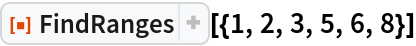Out=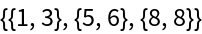### Scope (1)

The given list does not need to be sorted, but will be sorted automatically:

 In:=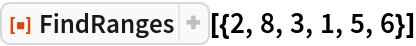Out=### Properties and Relations (1)

The returned ranges can be joined into the original set of numbers again:

 In:=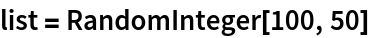Out=In:=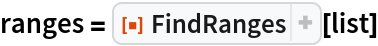Out=In:=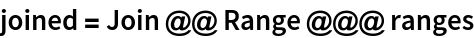Out=In:=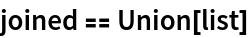Out=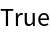### Possible Issues (2)

Noninteger numbers are "swallowed" if they are between consecutive integers in the list:

 In:=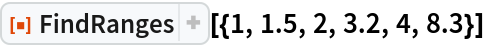Out=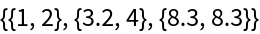Expressions that are not integers or real numbers each constitute their own "range":

 In:=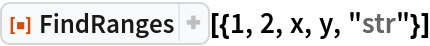Out=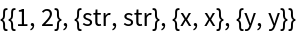Jan Poschko

## Requirements

Wolfram Language 11.3 (March 2018) or above

## Version History

• 1.0.0 – 04 February 2019